# How to Subtract in Google Sheets

At a very early stage in childhood, we were taught to subtract, and we learn it. Today we are living in an era that is fully computerized and digital. The calculations are very easy to do, and calculators have made this very simpler in our daily life, but subtracting values in Google sheets is much different; rather than subtracting a value by the calculator, the procedure is fully different. Many people are unaware of many things in Google Sheets and ask questions like how to subtract in Google Sheets and how to subtract columns in Google Sheets, etc. If you are one of those who is very curious to know how to do these calculations in Google Sheets, continue reading it, and you will be able to learn about the steps.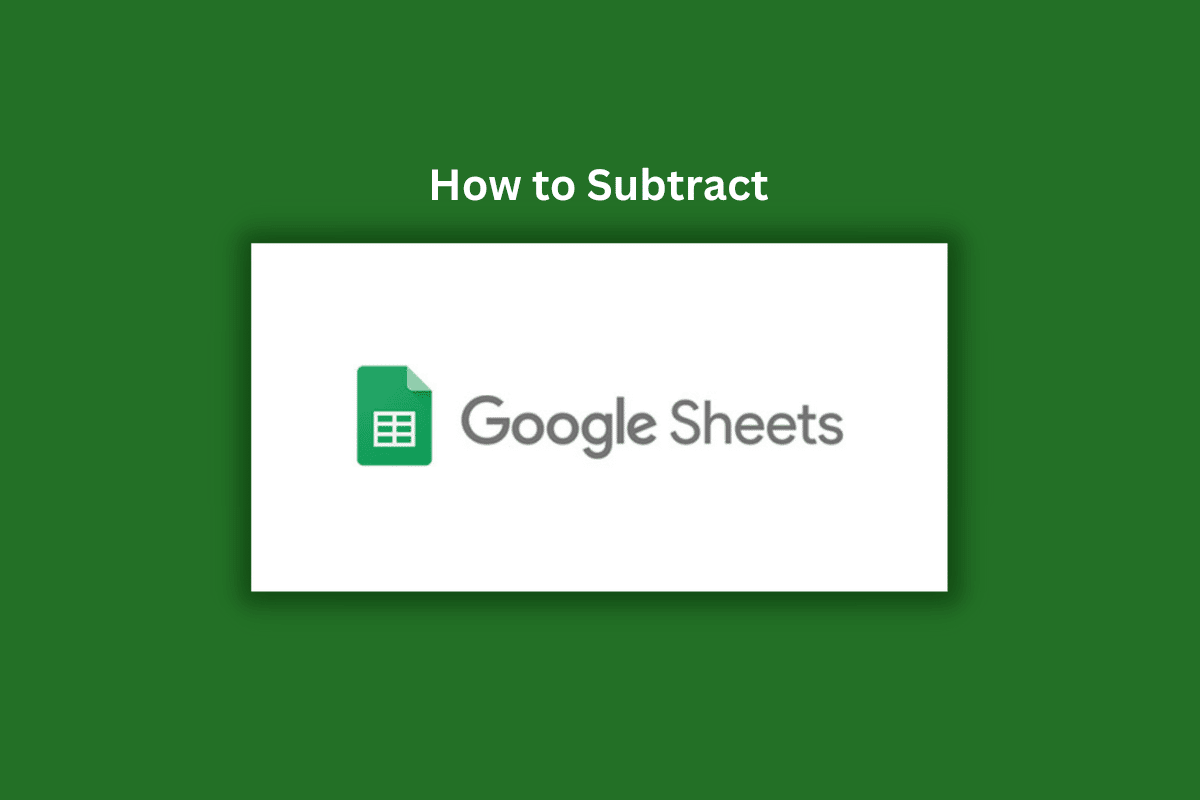## How to Subtract in Google Sheets

Read this article till the end to learn the steps demonstrating how to subtract in Google Sheets in a detailed manner using the pictures for better understanding.

### How to Subtract in Google Sheets using Simple Method?

Subtraction in Google Sheets is also very easy; you can subtract in many ways. Here we are discussing the simple method to subtract in Google Sheets using the = sign at first and putting the values as you did in calculators for subtraction. This formula is very simple to use and you need to enter only numeric values under this.

For example: =7− 4, The answer will be 3.

Hence, the subtraction is done with this easy method, but this can only be used at one time. For more calculations, you need to add a formula for subtracting any data values.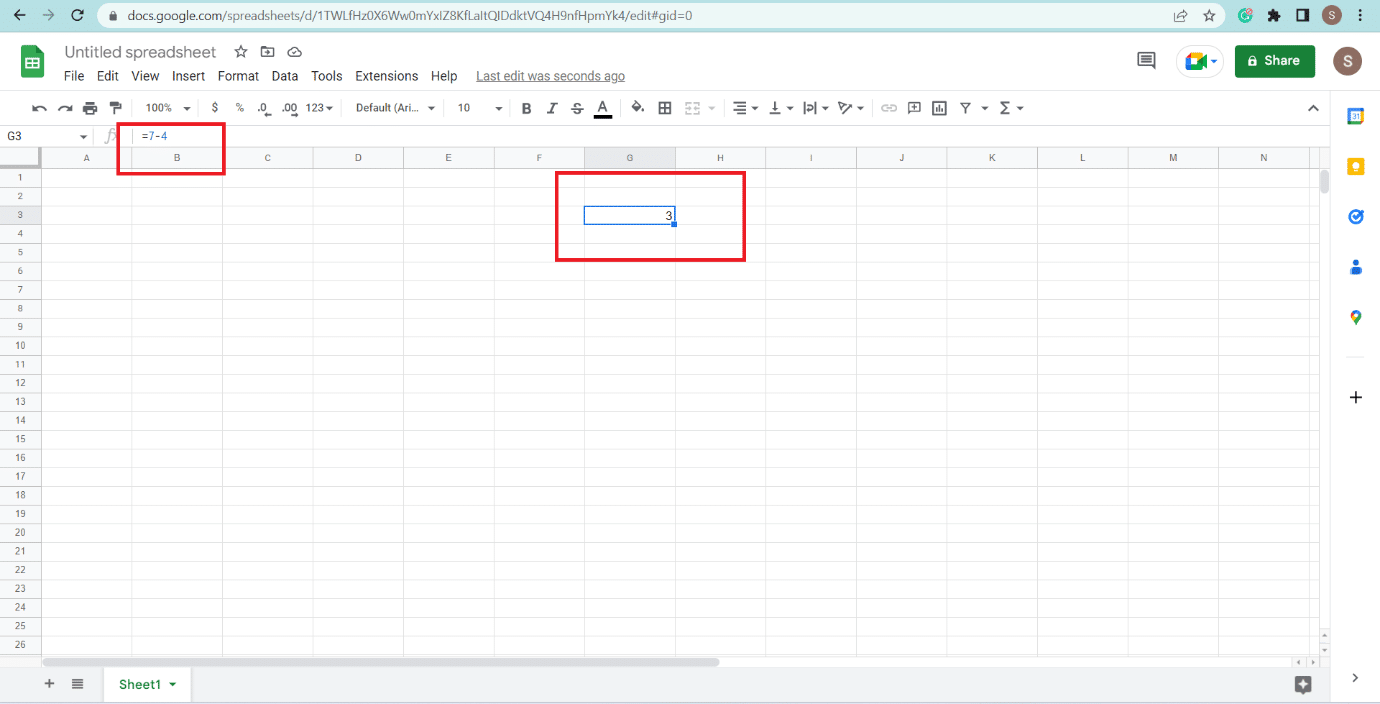### How to Subtract Columns in Google Sheets?

The subtraction of columns in Google Sheets is also very simple, but we need to put a formula for subtracting columns. This formula is used for long values, and you can drag the formula down if you want to use the same formula under your whole table. If you want to know the steps for how to subtract columns in Google Sheets, read below.

Note: Every formula in Google sheets starts with the = sign, and then the formula will be mentioned for any kind of subtraction, multiplication, addition, etc.

1. Type (=) sign in the desired cell where you wish to subtract columns on Google Sheets.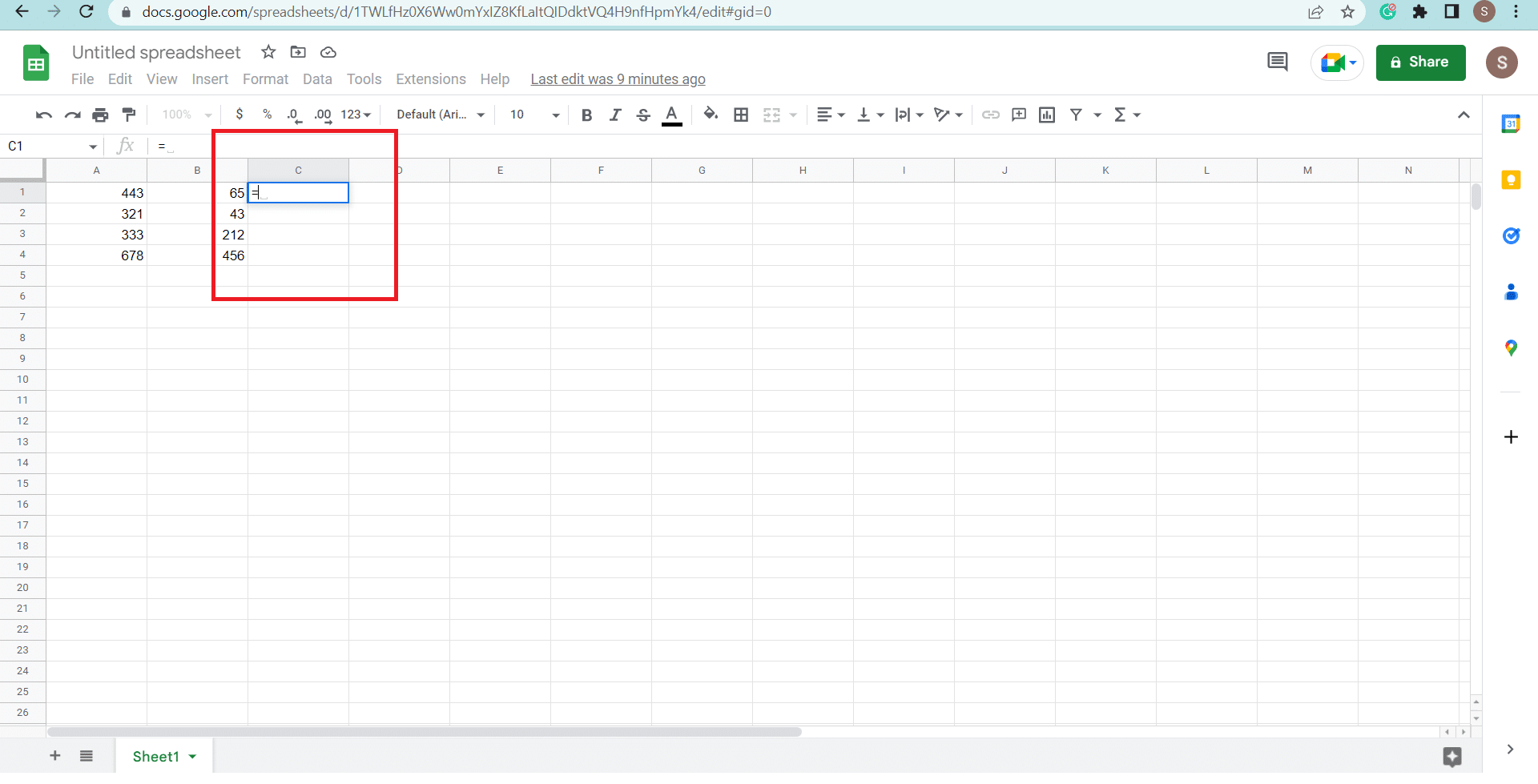2. Click on the first column from which you want the value subtracted.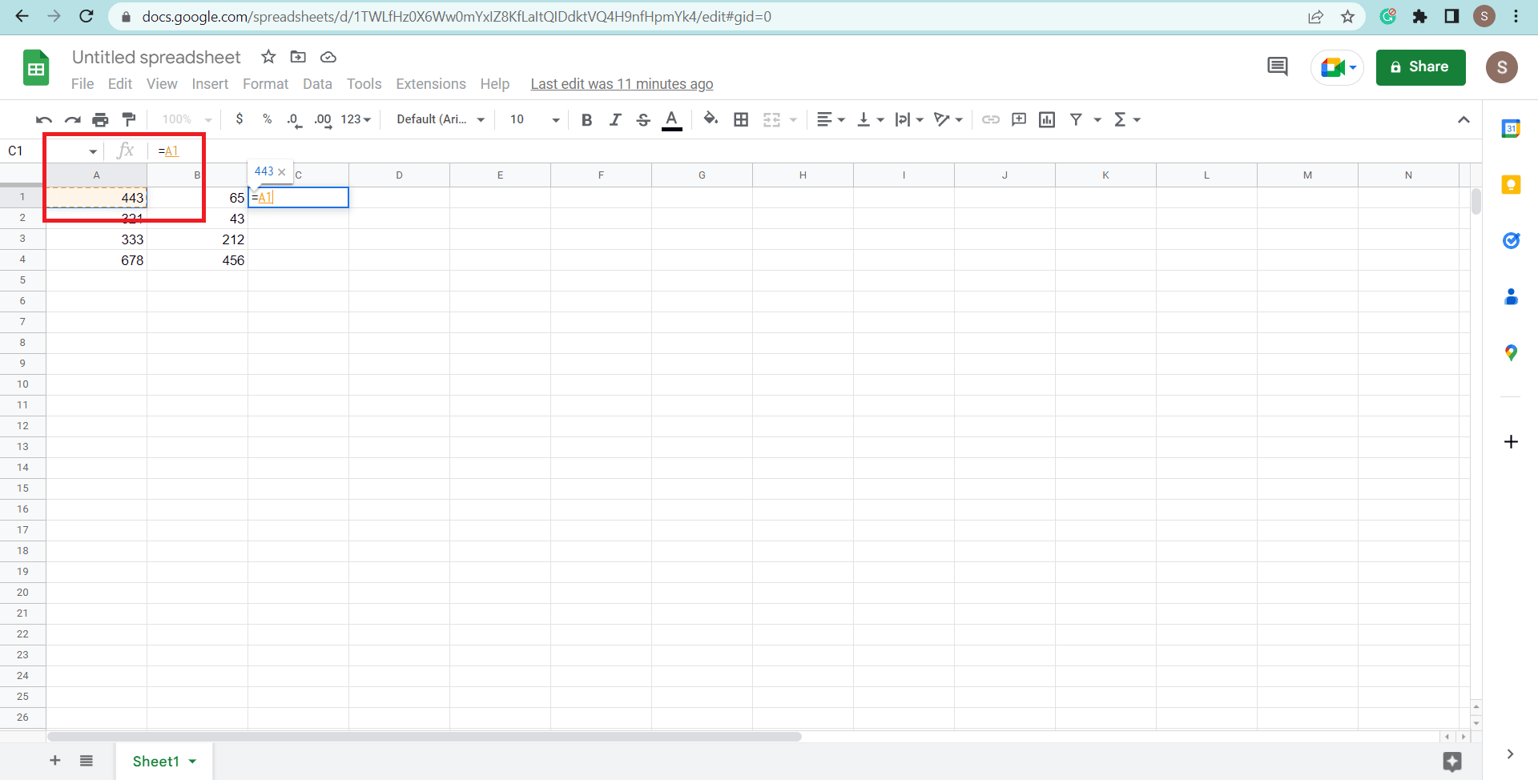3. Type the minus (-) sign.

4. Click on the next column.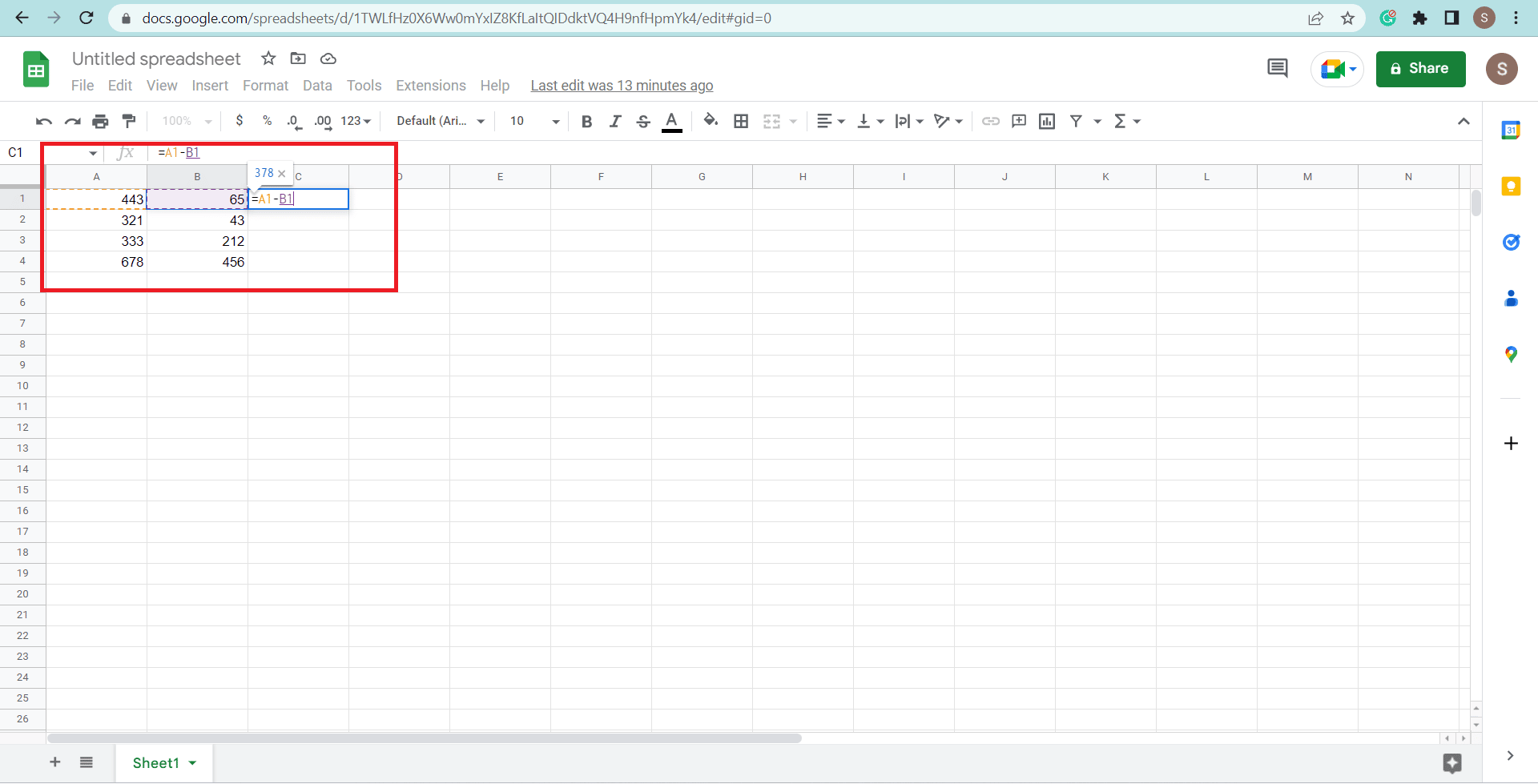5. Press the Enter key on your keyboard.

Note: If you want to subtract other columns with the same formula, select the cell where you have written the formula and drag down the select cursor in the corner of the cell. The remaining columns will get subtracted without using the formula again in each column.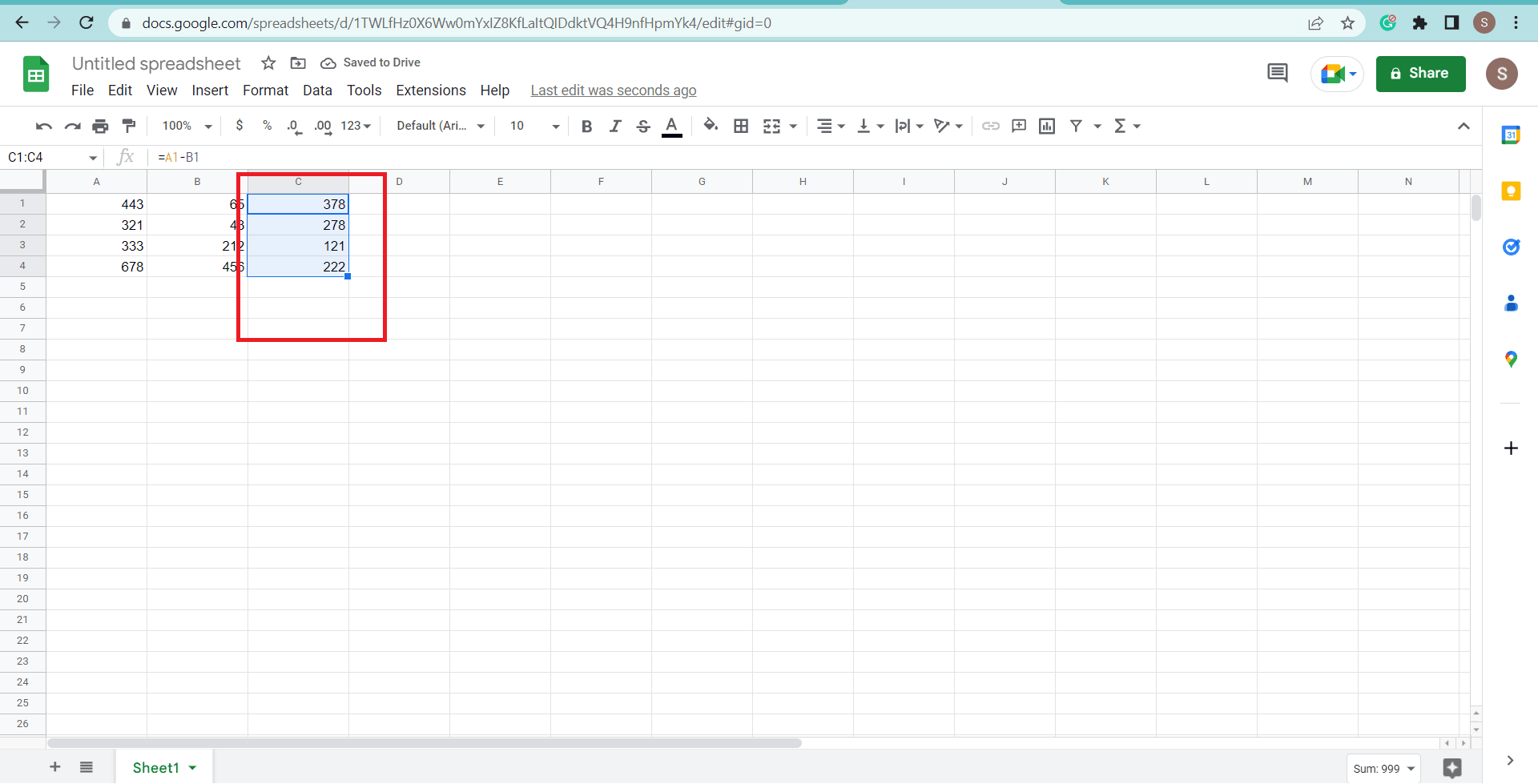This is how you can subtract the columns in Google Sheets with the easy and simple formula.

### How to Subtract Rows in Google Sheets?

Many people are confused about subtracting rows and they appear with the question of how to subtract rows in Google Sheets, as we did subtraction for columns, the same procedure and formula are used in rows also but what we need to do is we have to write and horizontally drag the formula, as for subtracting columns we vertically drag the formula. For more explanation about the subtraction of rows look at the steps.

1. Type the (=) sign below the row cell you want to subtract.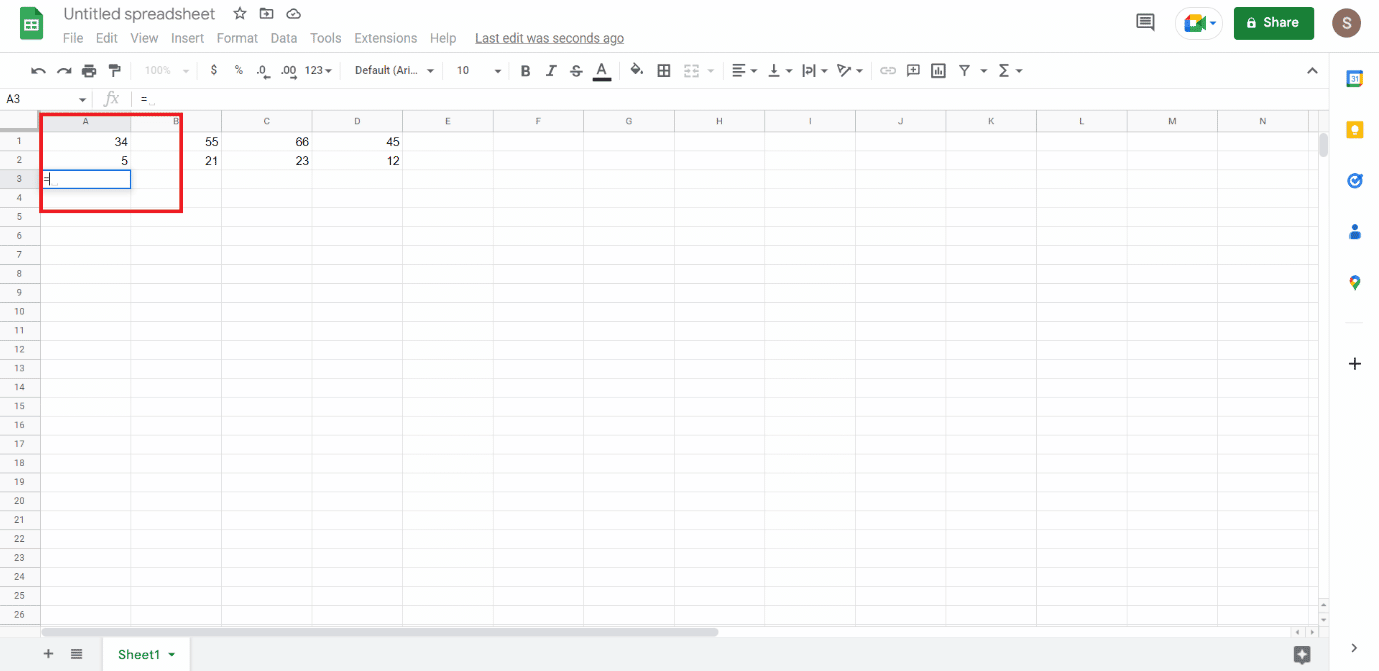2. Click on the row cell that you want to subtract.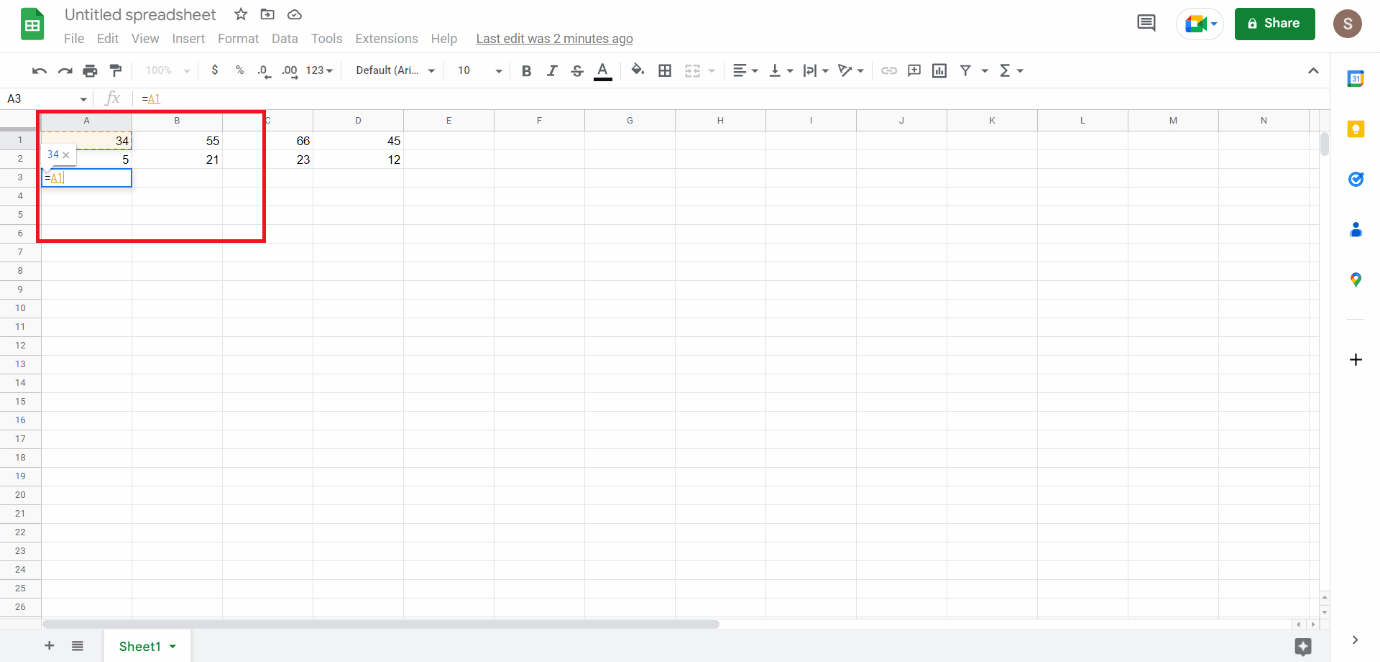3. Type minus (-) sign.

4. Click on another row cell.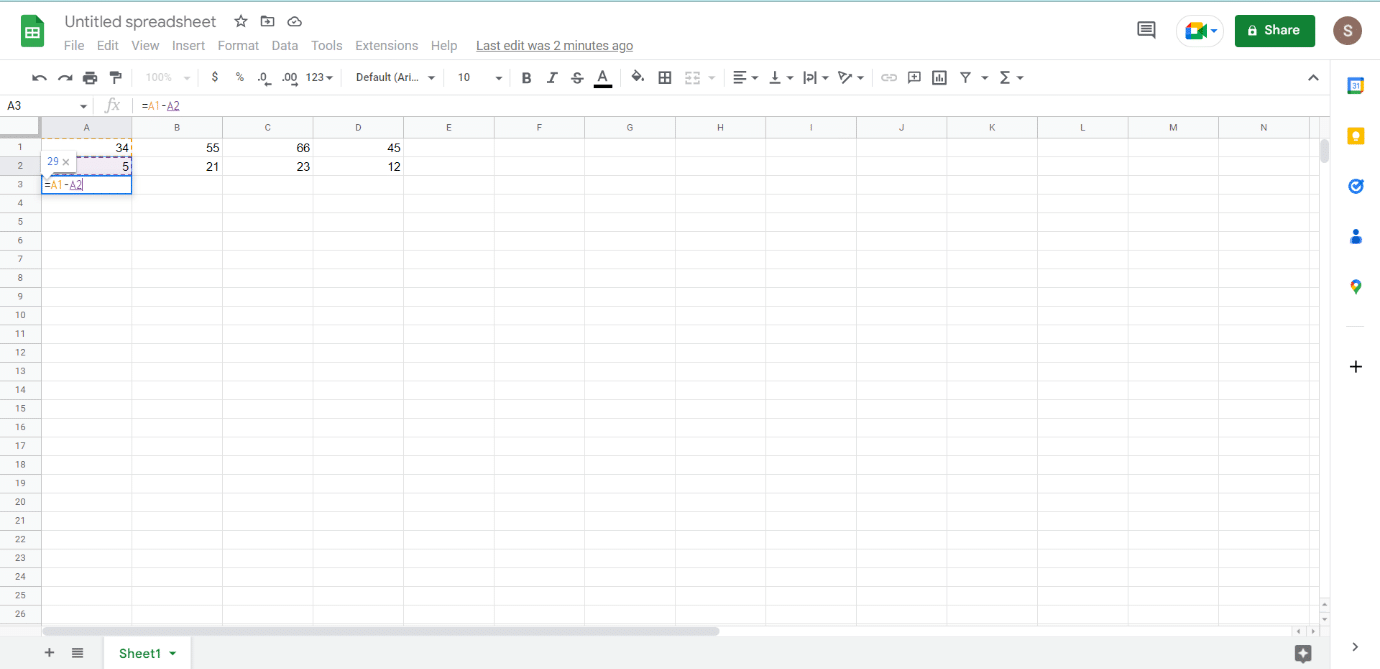5. Press Enter key.

6. Drag the answer cell horizontally if you want to subtract more rows with the same formula by selecting the cell and clicking on the + sign at the corner of the cell.### How to Subtract in Google Sheets Using the Minus Formula?

A formula is just a statement to make our calculation work, and a formula contains many values with operations like subtraction, addition, multiplication, division, etc. The values used in the formula can be word or numeric. When dealing with lots of data to be subtracted or calculated, it becomes hard to use a simple formula, so for this kind of subtraction, the formula is used in which minus text exits, and they can do all the subtraction easily. By using any formula, you can subtract many calculations in one go. Many people ask about how to do subtraction in google sheets by using the minus formula, so those who want to know can follow the steps.

2. Type the word MINUS.

3. Insert the Values in bracket ().4. Click on the desired cell you want for subtraction, like A1, A2, etc.

5. Insert Comma (,) at the end, as shown.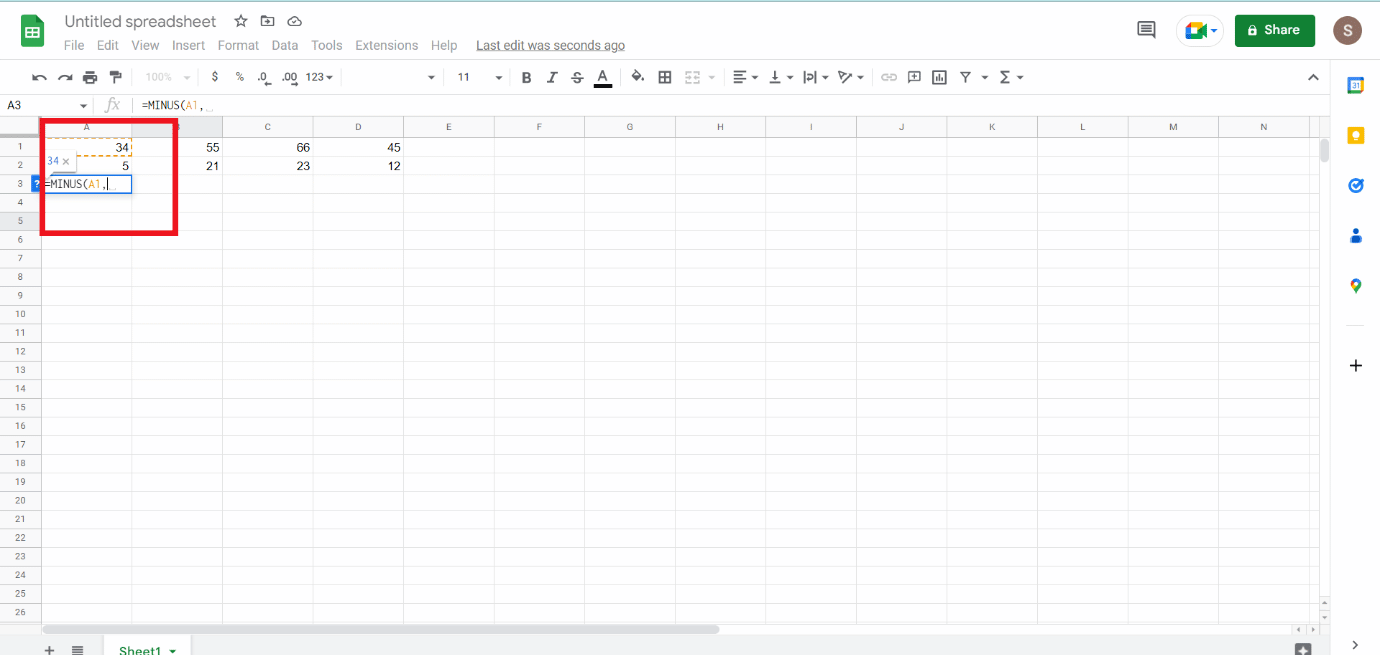6. Click on another cell for subtraction.7. Press Enter key.

If you want the same formula for other calculations also drag it where you want to know by selecting the cell and dragging it by + sign up to where you want to.

Recommended:

Here, we discussed the function and formula of subtraction in Google sheets and how to subtract rows and columns with the proper images to clarify the steps. We hope that this doc guided you on how to subtract in Google Sheets successfully. If you have any queries or suggestions, feel free to drop them in the comments section below.

#### How to Recover Messenger Account without Facebook

Jassweb always keeps its services up-to-date with the latest trends in the market, providing its customers all over the world with high-end and easily extensible internet, intranet, and extranet products.